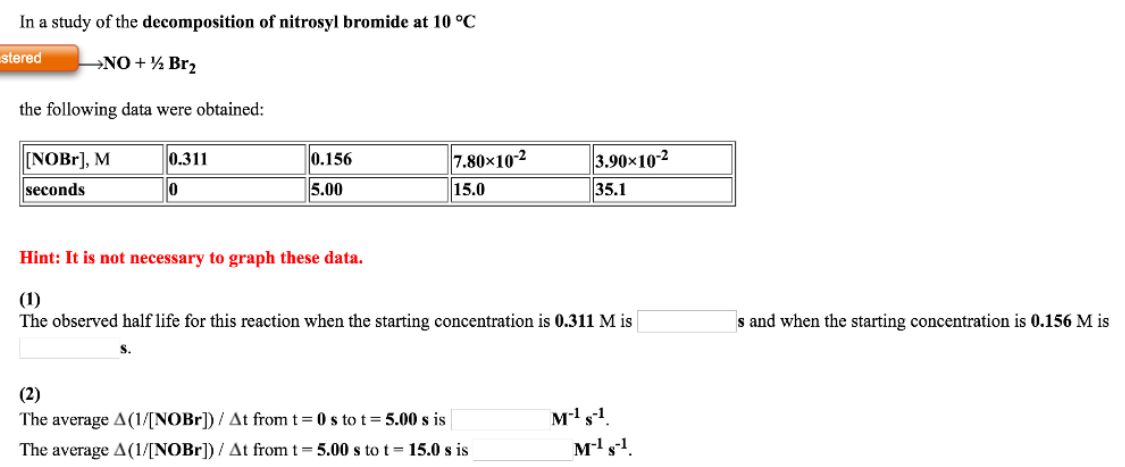# Problem: In a study of the decomposition of nitrosyl bromide at 10°C.NOBr → NO + 1/2 Br2 the following data were obtained: (1) The observed half life for this reaction when the starting concentration is 0.311 M is _________ s and when the starting concentration is 0.156 M is ________s. (2) The average Δ(1/[NOBr])/Δt from t = 0 s to t = 5.00 s is __________ M-1 s-1. The average Δ(1/[NOBr])/Δt from t = 5.00 s to t = 15.0 s is ___________M-1 s-1.

🤓 Based on our data, we think this question is relevant for Professor McVey's class at TXSTATE.

###### FREE Expert Solution###### Problem Details

In a study of the decomposition of nitrosyl bromide at 10°C.

NOBr → NO + 1/2 Br2

the following data were obtained:

(1) The observed half life for this reaction when the starting concentration is 0.311 M is _________ s and when the starting concentration is 0.156 M is ________s.

(2) The average Δ(1/[NOBr])/Δt from t = 0 s to t = 5.00 s is __________ M-1 s-1

The average Δ(1/[NOBr])/Δt from t = 5.00 s to t = 15.0 s is ___________M-1 s-1